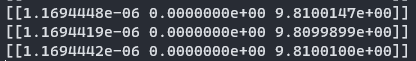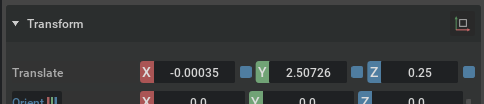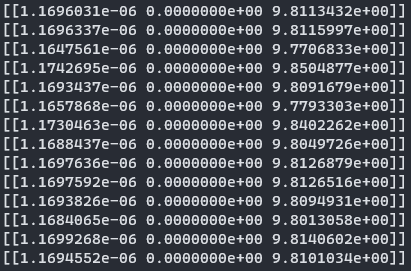# Is there a way to get friction forces?

I am obtaining contact forces using `RigidPrimView.get_net_contact_forces()`, but this appears to not include friction forces. Is there a way to get the friction force between a body that is in contact with the ground?

1 Like

Hi @jake.levy - In the Isaac Sim, the RigidPrimView.get_net_contact_forces() function provides the net contact forces on a rigid body, which includes both normal and friction forces. However, it does not provide a direct way to isolate the friction forces.

Friction forces are typically calculated internally within the physics engine based on the contact normal force, the friction coefficient of the materials in contact, and the relative velocity at the contact point. This information is not directly exposed through the Isaac Sim API.

If you need to estimate the friction force, you could potentially do so by making some assumptions and calculations. For example, if you know the total force, the normal force (which can be calculated from the weight of the object), and the angle of the surface, you could calculate the friction force using the equation:

Friction Force = Total Force - Normal Force * cos(Angle)

Please note that this is a simplification and may not accurately represent the actual friction force, especially in complex simulations with multiple contacts and dynamic conditions.

Hello @rthaker - thank you very much for the reply. However, I do not believe `RigidPrimView.get_net_contact_forces()` is giving friction forces. Here is a little experiment I did to check (but please let me know if I am doing something wrong).

I modified the Hello World isaac sim tutorial as follows:

• Set the mass of the cube to 1.0 kg
• Apply a physics material to the cube with static and dynamic coefficients of friction of 0.5
• Set the with static and dynamic coefficients of friction to 0.5 for the default ground plane
• Create a `RigidPrimView` of the cube
• Print the contact forces with `get_net_contact_forces()`

Here is the code:

``````from omni.isaac.examples.base_sample import BaseSample
from omni.isaac.core.objects import DynamicCuboid
import numpy as np
from omni.isaac.core.prims.rigid_prim_view import RigidPrimView
from omni.isaac.core.materials.physics_material import PhysicsMaterial

# Note: checkout the required tutorials at https://docs.omniverse.nvidia.com/app_isaacsim/app_isaacsim/overview.html

class HelloWorld(BaseSample):
def __init__(self) -> None:
super().__init__()
return

def setup_scene(self):

world = self.get_world()
dynamic_friction=0.5)
DynamicCuboid(
prim_path="/World/random_cube",
name="fancy_cube",
position=np.array([0, 0, 1.0]),
scale=np.array([0.50, 0.50, 0.50]),
color=np.array([0, 0, 1.0]),
mass=1.0,
physics_material=PhysicsMaterial(
"/World/Physics_Materials/cube_mat",
name="cube_mat",
static_friction=0.5,
dynamic_friction=0.5
)
))
self._cube_contact = RigidPrimView(
prim_paths_expr="/World/random_cube",
name="cube_vew",
reset_xform_properties=False,
track_contact_forces=True,
prepare_contact_sensors=True,
)
return

self._world = self.get_world()
self._cube = self._world.scene.get_object("fancy_cube")
return

def print_cube_info(self, step_size):
print(self._cube_contact.get_net_contact_forces(dt=step_size))

async def setup_pre_reset(self):
return

async def setup_post_reset(self):
return

def world_cleanup(self):
return
``````

Now, when I load the cube in, start the sim, and let it fall to the ground, the reported contact forces are close to [0.0, 0.0, 9.81] N, which is expected for the cube at rest on the ground:Next, with the simulation paused, I will set the y-axis linear velocity of the `random_cube` to 5 m/s:Now, basic physics calculations assuming m=1.0 kg; mu=0.5; g=9.81 ms^-2; v0 = 5.0 m/s result in a change in y of 2.55 m. I let the simulation play out until the cube comes to rest and look at the y-position of the cube:The cube stops at 2.507 m; close to our guess of 2.55 m. This should validate that friction forces are happening to the cube with a coefficient of dynamic friction of 0.5.

However, when I examine the output from `get_net_contact_forces()` while the cube was moving during the experiment, the contact force in the y-direction is still zero, while the expected force should be (mu)(m)(g) = 4.9 N:This is what leads me to believe that friction is not being reported as part of the contact forces.

@jake.levy
You are correct. The current behavior of the `get_net_contact_forces` and other contact APIs do not include any frictional effects. We are in the process of including frictional components in the API for the upcoming Isaac sim release.